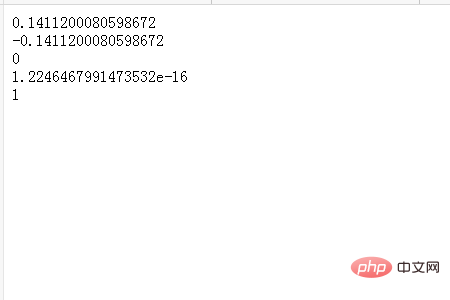### sin方法怎么使用

JavaScript中的sin()方法可返回一个数字的正弦，所以接下来的这篇文章我们就来具体看看sin()方法的使用。`Math.sin(x)`

x表示一个以弧度表示的角。将角度乘以 0.017453293 （2PI/360）即可转换为弧度。

```<!DOCTYPE html>
<html>
<body>
<script type="text/javascript">
document.write(Math.sin(3) + "<br />")
document.write(Math.sin(-3) + "<br />")
document.write(Math.sin(0) + "<br />")
document.write(Math.sin(Math.PI) + "<br />")
document.write(Math.sin(Math.PI/2))
</script>
</body>
</html>```### Erlo大厅()

* 这里是“吐槽厅”，所有人可看，只保留当天信息。

• ###Erlo.vip2019-08-21 05:34:00Hello、欢迎使用吐槽厅，这里是个吐槽的地方。
• 首页 笔记分享 案例展示 ERLO 搜索
鼠标试试
返回顶部